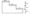# CSVTU Exam Papers – BE I Year –Engineering Graphics– April-May-2010

BE (1st Year)

Examination, Nov-Dec-  2009-10

Engineering Graphics

Q. 1 .(a) What are the different types of scales ?

(b) On a map,, the distance between two points is 14 cm. The real distance between them is 20 km. Draw a diagonal scale this map to read kilometers and hectameters and to measure upto 25 km Show a distance of 17.6 km on this scale.

(c) Two points A and B are 50 mm apart. Draw the curve traced out by a point P moving in such a way that the difference between its distances from A and B is always constant and equal to 20 mm.

(d) A circle of 50 mm diameter rolls on the circumference of: another.circle of 175 mm diameter and outside it. Trace the locus of a point on the circumference of the rolling circle for one complete revolution.

Q.2.(a) What is orthographic projection ?

(b) The -length of the top view of a line parallel to the V.P.-and inclined to’45° to the H.P. is 5 cm one end of the line Is 1.2 cm above the H.P. and 2.5 cm infront of the V.P. Draw the projections of the line and determine its “true length.

(c) A line AB 50 mm long has its end A in both the H.P. and the V.P. it is inclined at 30° to the H.P. and at 45°, to the V.P. Draw its projections.

(d) The top view of a 75 mrn long line AB measures 65 rim vvhile* the length of its front view is 50 mm its one end A is in the H.P. and, 12 rnrrf infront of the V.P. Draw its projection of AB andi determine its inclination with H.P. and the V.P.

Q.3.(a) What are the different types of auxiliary planes ?

(b) An isosceles triangle PQR having the sides of 75 mm long and altitude 75 mm has its corners P, Q and R 25 mm, 50 mm and 75 mm respectively above the ground. Draw its: projection.

(c) Draw the projection of a hexagonal pyramid base 30mm side and axis 50 mm long, having its base on the HP and on the edge of the base inclined at 45 to the V.P.

(d) A triangular prism, base 30-mm side and axis 50 ‘m|n long lying on the H.P. on one of its rectangular faces with it s axis inclined at 30° to the V.P. it is cut by a horizontal sec at a distance of 12 mm above the ground. Draw its and sectional top view.

Q. 4 (a) What is development ?

(b) Draw the development of the lateral surfacje P of cylinder shown in Fig.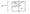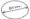(c) Draw the isometric view of the casting shown in two views :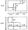(d) Draw the isometric projection of a sphere, resting centrally on the top of a square prism, the front view of which is shown :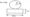(b) What are the benefits of CAD and limitations?

(c) Explain the function of commands with the help of example.

(i) COPY

(ii) ROTATE

(iii) STRETCH

(iv) SCALE

(v) TRACE

(vi) FILL

(vii) OFFSET

(d) Write the method by AutoCAD to draw the following sketch using :

(i) Absolute Coordinate and

(ii) Polar coordinate system.

Fig:-